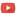KE8QZC | SFW | TSWSyllabus: [pdf] [tex]

Exams
Exam 1 [pdf] [tex]
Exam 2 [pdf] [tex]
Exam 3 [pdf] [tex]

Homework
Homework 1 (due 24 January) (solution: [pdf] [tex]): Chapter 1: Read #0. Do #1, 2, 6, and write proofs for the two unproven claims that we discussed in Friday's class. (note: those claims are the claim that the pair $C|D$ defined in Theorem 2 is a cut and the claim that $C|D$ is an upper bound for $\mathscr{C}$).
Homework 2 (due 31 January) (solution): Chapter 1: #8(a), 11 (don't need to use cuts), and the following problems:
Problem A: Prove from the definition for convergence that $\displaystyle\lim_{n \rightarrow \infty} \dfrac{1}{n}=0$.
Problem B: Prove from the definition for convergence that $\displaystyle\lim_{n \rightarrow \infty} \dfrac{n}{2n+1} = \dfrac{1}{2}$.
Problem C: Prove from the definition for convergence that $\displaystyle\lim_{n \rightarrow \infty} \dfrac{3n+2}{5n-1} = \dfrac{3}{5}$.
Homework 3 (due 12 February) (solution: [pdf] [tex]): [pdf] [tex]
Homework 4 (due 21 February) (solution: [pdf] [tex]): [pdf] [tex]
Homework 5 (due 28 February) (solution: [pdf] [tex]): [pdf] [tex]
Homework 6 (due 9 March) (solution: [pdf] [tex]) : [pdf] [tex]
Homework 7 (due 19 March) (solution: [solution): [pdf] [tex]
Homework 8 (due 2 April) (solution: [pdf] [tex]): [pdf] [tex]
Homework 9 (due 11 April) (solution: [pdf] [tex]): [pdf] [tex]
Homework 10 (due 18 April) (solution): [pdf] [tex]
Homework 11 (due 25 April) (solution): [pdf] [tex]
Homework 12 (due by last day of finals) (solution): [pdf] [tex]

Quizzes
Quiz 1: (solution)
Quiz 2: (solution)
Quiz 3: (solution)
Quiz 4: (solution)
Quiz 5: (solution)
Quiz 6: (solution)
Quiz 7: (solution)
Quiz 8: (see 9 April slides)
Quiz 9: (see 9 April slides)
Quiz 10: (see 11 April slides)
Quiz 11: (solution)
Quiz 12: (solution)
Quiz 13: (solution)
Quiz 14: (solution)

Slides
26-28 March, 2 April 2018: [pdf] [tex]
4 April: [pdf]
9 April: [pdf] [tex]
11 April: [pdf] [tex]
13 April: [pdf] [tex]
16 April: [pdf] [tex]
23 April: [pdf] [tex]
25 April: [pdf] [tex]
27 April: [pdf] [tex]
Notes
24 January 2018: convergence proofs [pdf] [tex]
9 February 2018: continuity of a quadratic [pdf] [tex]
Study topics for exam 1: [pdf] [tex]
Study topics for exam 2: [pdf] [tex]
Study topics for exam 3: [pdf] [tex]
Study topics for final: [pdf] [tex]